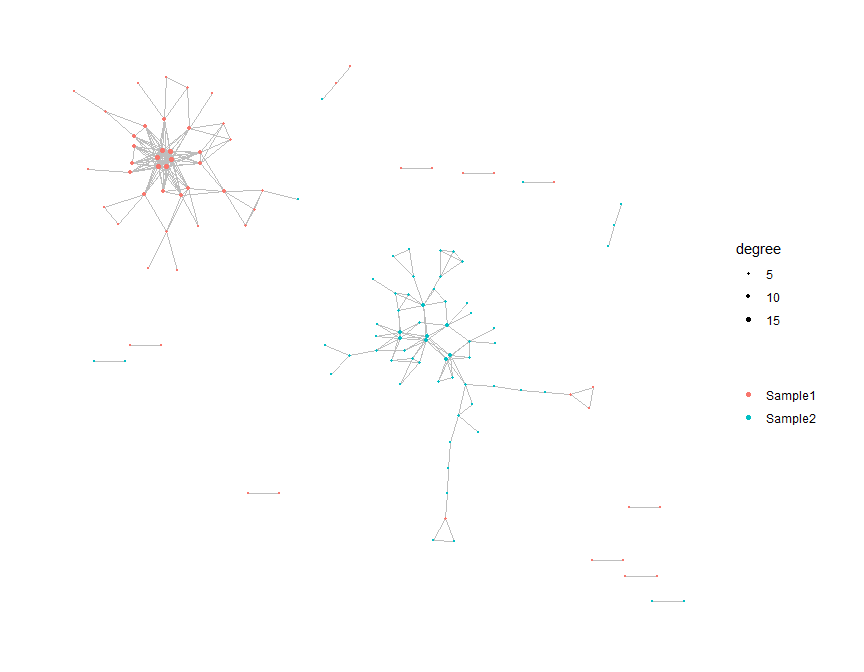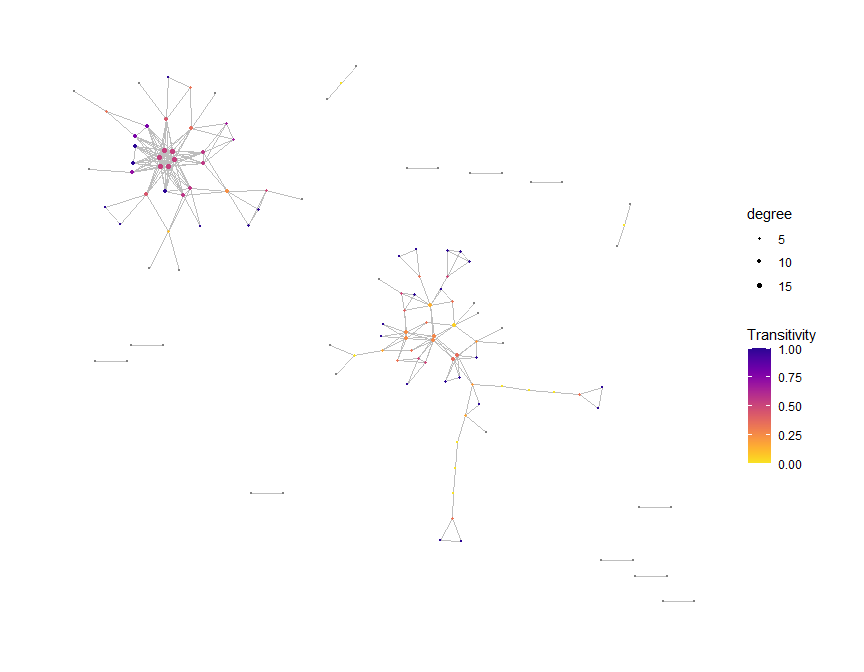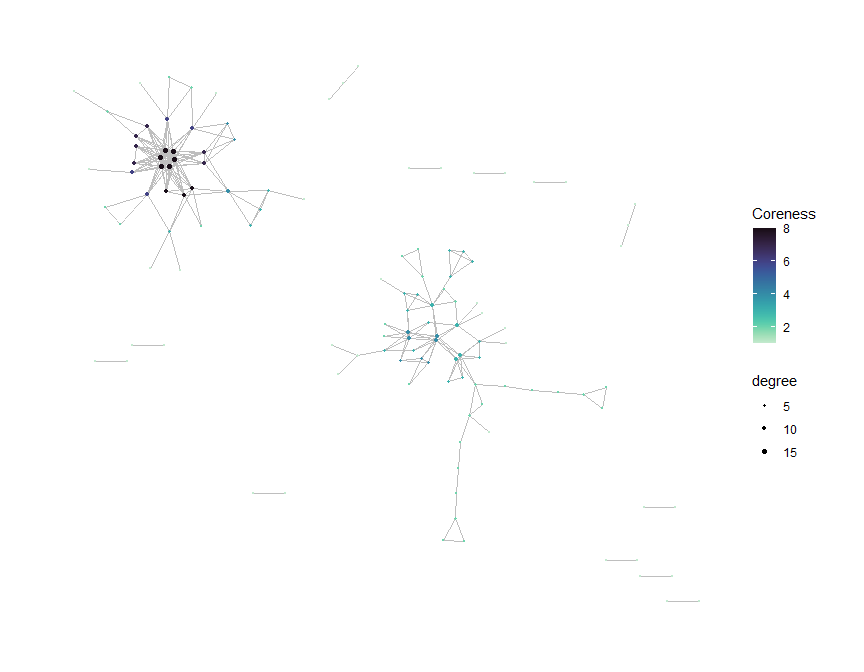# Introduction

General network analysis on Adaptive Immune Receptor Repertoire Sequencing (AIRR-Seq) data is performed using buildRepSeqNetwork() or its convenient alias buildNet(). This function does the following:

• Filters the AIRR-Seq data according to user specifications
• Builds the network graph for the immune repertoire
• Performs additional network analysis, which can include:
• Cluster analysis
• Network properties
• Customizable visual plots of the network graph
• Returns (and optionally saves) the following output:
• The network graph (as igraph and adjacency matrix)
• Metadata for the nodes in the network
• Metadata for the clusters in the network
• Plots of the network graph

#### Simulate Data for Demonstration

We simulate some toy data for demonstration.

We simulate data consisting of two samples with 100 observations each, for a total of 200 observations (rows).

set.seed(42)
library(NAIR)
#> Welcome to NAIR: Network Analysis of Immune Repertoire.
#> Get started using vignette("NAIR"), or by visiting
#> https://mlizhangx.github.io/Network-Analysis-for-Repertoire-Sequencing-/
dir_out <- tempdir()

toy_data <- simulateToyData()
#>        CloneSeq CloneFrequency CloneCount SampleID
#> 1 TTGAGGAAATTCG    0.007873775       3095  Sample1
#> 2 GGAGATGAATCGG    0.007777102       3057  Sample1
#> 3 GTCGGGTAATTGG    0.009094910       3575  Sample1
#> 4 GCCGGGTAATTCG    0.010160859       3994  Sample1
#> 5 GAAAGAGAATTCG    0.009336593       3670  Sample1
#> 6 AGGTGGGAATTCG    0.010369470       4076  Sample1
nrow(toy_data)
#>  200

# Required Arguments

• The first parameter data accepts a data frame containing the AIRR-seq data, where each row corresponds to a single TCR/BCR clone (bulk data) or cell (single-cell data).
• The second parameter seq_col specifies the data column containing the receptor sequences to be used as the basis of similarity between two cells or clones. It accepts the column name as a character string or the column position index.
net <- buildNet(toy_data, "CloneSeq")

# Filtering the Input Data

The following options are useful for removing noise or irrelevant data from the analysis, potentially improving the quality of the network graph and downstream analysis results.

#### Sequence Length

The min_seq_length parameter specifies the minimum TCR/BCR sequence length (in number of characters). Sequences with lengths below this value are dropped prior to analysis. An argument value of NULL bypasses this filter. This filter applies to the sequence in the column specified by seq_col.

By default, min_seq_length is set to 3.

net <- buildNet(toy_data, "CloneSeq", min_seq_length = 10)

#### Sequence Content

The optional drop_matches parameter can be used to remove TCR/BCR sequences matching a specified pattern. It accepts a character string containing a regular expression specifying the pattern of content to search for. Each sequence in the column specified by seq_col is checked for a pattern match using grep(). If a match is found, the sequence is removed prior to analysis.

net <- buildNet(toy_data, "CloneSeq", drop_matches = "\\W")

# Network Settings

The following settings pertain to the network construction.

#### Distance Function

The default method for quantifying the similarity between TCR/BCR sequences is the Hamming distance, which is computed using hamDistBounded(). It calculates the number of differences between two sequences of the same length. For each position in one sequence, the character in that position is checked to see whether it differs from the character in the same position of the other sequence. If the sequences have different lengths, the shorter sequence is extended by adding non-matching characters to make it the same length as the longer sequence.

The Levenshtein distance, which is computed using levDistBounded(), can be used as an alternative measurement to determine the similarity between sequences. It calculates the minimum number of single-character edits (insertions, deletions and transformations) needed to transform one sequence into the other. This method is particularly useful for comparing sequences of different lengths and can account for insertions and deletions. When constructing a network based on the similarity of CDR-3 nucleotide sequences, it is preferable to use the Levenshtein distance instead of the default Hamming distance by specifying dist_type = "lev". However, the Levenshtein distance requires significantly more computation than the Hamming distance, which may be challenging when working with large data sets having long TCR/BCR sequences.

net <- buildNet(toy_data, "CloneSeq", dist_type = "lev")

#### Distance Cutoff

The function specified by dist_type quantifies the similarity between TCR/BCR sequences as a nonnegative distance, with values closer to 0 indicating greater similarity.

Each node in the network graph corresponds to a row of the AIRR-Seq data. By default, two nodes are connected by an edge if the distance between their TCR/BCR sequences does not exceed 1. This cutoff value is specified by the dist_cutoff parameter, which controls the stringency of the network construction and affects the number and density of edges in the network. A lower cutoff requires greater sequence similarity to form an edge connection. If dist_cutoff = 0, two sequences must be identical in order for their nodes to be joined by an edge.

net <- buildNet(toy_data, "CloneSeq", dist_cutoff = 0)

#### Keep/Remove Isolated Nodes

By default, only nodes that are joined by an edge connection to at least one other node will be kept in the network.

If drop_isolated_nodes = FALSE, then all nodes are kept in the network, including those that do not have any edge connections to other nodes.

net <- buildNet(toy_data, "CloneSeq", drop_isolated_nodes = FALSE)

# Network Properties and Cluster Analysis

buildRepSeqNetwork() can perform additional analysis after constructing the network, including cluster analysis (partitioning the network graph into densely-connected subgraphs) and computation of network properties (which describe the structural organization of the network).

### Node-Level Network Properties

Node-level network properties pertain to individual nodes in the network graph.

Some are local properties, meaning that their value for a given node depends only on a subset of the nodes in the network. One example is the network degree of a given node, which represents the number of other nodes that are directly joined to the given node by an edge connection.

Other properties are global properties, meaning that their value for a given node depends on all of the nodes in the network. An example is the authority score of a node, which is computed using the entire graph adjacency matrix (if we denote this matrix by $$A$$, then the principal eigenvector of $$A^T A$$ represents the authority scores of the network nodes).

Node-level network properties can be computed when calling buildRepSeqNetwork() by setting node_stats = TRUE, or as a separate step using addNodeStats().

net <- buildNet(toy_data, "CloneSeq", node_stats = TRUE)

See this vignette for more details on computing node-level network properties.

### Cluster Analysis

Cluster analysis uses a community-finding algorithm to partition the network graph into clusters (densely-connected subgraphs). These clusters represent groups of clones/cells with similar receptor sequences.

Cluster analysis can be performed when calling buildRepSeqNetwork() by setting cluster_stats = TRUE or as a separate step using addClusterStats().

net <- buildNet(toy_data, "CloneSeq", cluster_stats = TRUE)

The cluster membership of each node is recorded as a variable in the node metadata. Cluster properties, such as node count and mean sequence length, are included in their own data frame within the output.

See this vignette for more details.

# Visualization

Customized plots can be produced when calling buildRepSeqNetwork() or created afterward using addPlots().

Specify print_plots = TRUE to print the plots to the R plotting window.

net <- buildNet(toy_data, "CloneSeq",
node_stats = TRUE,
color_nodes_by = c("SampleID", "transitivity", "coreness"),
color_scheme = c("default", "plasma-1", "mako-1"),
color_title = c("", "Transitivity", "Coreness"),
size_nodes_by = "degree",
node_size_limits = c(0.1, 1.5),
plot_title = NULL,
plot_subtitle = NULL,
print_plots = TRUE
)# Output

The function returns a list with the following elements:

names(net)
#>  "details"          "igraph"           "adjacency_matrix" "node_data"
#>  "plots"

We describe each element below.

details records argument values supplied in the call to buildRepSeqNetwork():

net$details #>$seq_col
#>  "CloneSeq"
#>
#> $dist_type #>  "hamming" #> #>$dist_cutoff
#>  1
#>
#> $drop_isolated_nodes #>  TRUE #> #>$nodes_in_network
#>  122
#>
#> $min_seq_length #>  3 #> #>$drop_matches
#>  "NULL"

nodes_in_network records the number of nodes in the network. Additional network information is included when cluster analysis is performed.

node_data is a data frame containing metadata for the network nodes, where each row corresponds to a node in the network graph:

head(net$node_data) #> CloneSeq CloneFrequency CloneCount SampleID degree transitivity #> 2 GGAGATGAATCGG 0.007777102 3057 Sample1 1 NaN #> 5 GAAAGAGAATTCG 0.009336593 3670 Sample1 3 0.3333333 #> 8 GGGGAGAAATTGG 0.006220155 2445 Sample1 2 1.0000000 #> 11 GGGGGAGAATTGC 0.012969469 5098 Sample1 4 0.6666667 #> 12 GGGGGGGAATTGC 0.009079646 3569 Sample1 10 0.3555556 #> 13 AGGGGGAAATTGG 0.014941093 5873 Sample1 5 0.1000000 #> eigen_centrality centrality_by_eigen betweenness centrality_by_betweenness #> 2 0.00000000 0.00000000 0.000000 0.000000 #> 5 0.00000000 0.00000000 108.000000 108.000000 #> 8 0.04558649 0.04558649 0.000000 0.000000 #> 11 0.15055366 0.15055366 1.549451 1.549451 #> 12 0.52691798 0.52691798 73.970918 73.970918 #> 13 0.14682343 0.14682343 75.439560 75.439560 #> authority_score coreness page_rank #> 2 0.00000000 1 0.008196721 #> 5 0.00000000 2 0.010578507 #> 8 0.04558649 2 0.003936684 #> 11 0.15055366 4 0.005034736 #> 12 0.52691798 6 0.011491588 #> 13 0.14682343 3 0.008703523 Since buildNet() was called with drop_isolated_nodes = TRUE, some of the original rows are missing. The original row names are preserved, facilitating cross-referencing with the original data. By default, all variables from the original input data are present. To include only a subset of the original variables, specify those to keep using the subset_cols parameter, which accepts a character vector of column names or a vector of column indices. The sequence column is always included. Variables for node-level network properties will also be present if computed, as seen here. Variables present in the node metadata can be used to encode node colors in visualizations. #### Cluster Metadata If buildRepSeqNetwork() is called with cluster_stats = TRUE, the returned list will contain a data frame cluster_data with cluster properties such as node count and mean sequence length, as seen here. #### Visual Plots plots is a list containing any plots created as well as a matrix graph_layout storing the node layout used in the plots. Each plot is named according to the variable used to color the nodes. names(net$plots)
#>  "SampleID"     "transitivity" "coreness"     "graph_layout"

Each plot is a ggraph (a special kind of ggplot).

class(net$plots$uniform_color)
#>  "NULL"

Custom plots can be created when calling buildNet() or afterward using addPlots() as described here.

#### Network Graph and Adjacency Matrix

igraph is an object of class igraph and adjacency_matrix is a dgCMatrix. Both objects encode the nodes and edges of the network graph, each using a different format. These objects are used by other NAIR functions, and are typically not of direct importance to the user.

# Saving Output

#### Output Directory

buildRepSeqNetwork() writes its output to files if a directory path is provided to the output_dir parameter. The specified output directory will be created if it does not already exist.

#### Output File Format

• By default, the list returned by buildRepSeqNetwork() is saved as a compressed RDS file.
• If the file will be transferred across machines, the RData format is preferred. It can be used by specifying output_type = "rda". The list will be named net in the R environment.
• To use the results outside of R, specify output_type = "individual". Each list element will be saved as a separate file using the following formats:
• .csv for node_data and cluster_data (using write.csv(), with row.names = FALSE for cluster_data)
• .mtx for adjacency_matrix
• .txt for details, igraph and plots$graph_layout • .rda (RData) for plots • Regardless of output_type, plots are printed to a pdf file containing one plot per page. The dimensions (in inches) for each page can be adjusted using the plot_width and plot_height parameters, with the defaults being 12 and 10, respectively. #### Output File Name(s) By default, the name of each saved file begins with MyRepSeqNetwork. This prefix can be changed using the output_name parameter, which accepts a character string. When output_type is "rds" or "rda", only two files are saved (the data file and the pdf). For each file, output_name is followed by the appropriate file extension. For example, if output_name = "NetworkABC" and output_type = "rds", then the files will be named NetworkABC.rds and NetworkABC.pdf. • If output_type = "individual", the prefix specified by output_name is followed by: • _Details.txt for details • _EdgeList.txt for igraph • _AdjacencyMatrix.mtx for adjacency_matrix • _NodeMetadata.csv for node_data • _ClusterMetadata.csv for cluster_data (if present) • _Plots.rda for the list plots • .pdf for the pdf of the plots • _GraphLayout.txt for plots$graph_layout

For example, if output_name = "NetworkABC" and output_type = "individual", then the node metadata is saved as NetworkABC_NodeMetadata.csv, the pdf is saved as NetworkABC.pdf, the list plots is saved as NetworkABC_Plots.rds, and so on.

# Supplementary Functions

The NAIR package contains a number of functions supplementary to buildRepSeqNetwork() that can be used to perform additional downstream tasks. See this vignette.

library(magrittr) # For pipe operator (%>%)
toy_data %>%
filterInputData("CloneSeq", drop_matches = "\\W") %>%
buildNet("CloneSeq") %>%
saveNetwork(output_dir = tempdir(), output_name = "my_network")